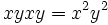# Square map is endomorphism iff abelian

(diff) ← Older revision | Latest revision (diff) | Newer revision → (diff)
Jump to: navigation, search

## Contents

This article describes an easy-to-prove fact about basic notions in group theory, that is not very well-known or important in itself
View other elementary non-basic facts
VIEW FACTS USING THIS: directly | directly or indirectly, upto two steps | directly or indirectly, upto three steps|
VIEW: Survey articles about this

## Statement

### Verbal statement

The square map on a group, viz the map sending each element to its square, is an endomorphism if and only if the group is Abelian.

### Symbolic statement

Let$G$ be a group and$\sigma:G \to G$ be the map defined as$\sigma(x) = x^2$. Then,$\sigma$ is an endomorphism if and only if$G$ is Abelian.

## Proof

### From endomorphism to Abelian

Suppose$\sigma = x \mapsto x^2$ is an endomorphism of the group$G$. Then for any$x,y \in G$ we want to show that$x$ and$y$ commute. This can be proved as follows:$\sigma(xy) = \sigma(x)\sigma(y)$ becaus$\sigma$ is an endomorphism

Thus:$xyxy = x^2y^2$

Cancelling the leftmost$x$ and the rightmost$y$, we get:$yx = xy$

and hence$x,y$ commute.